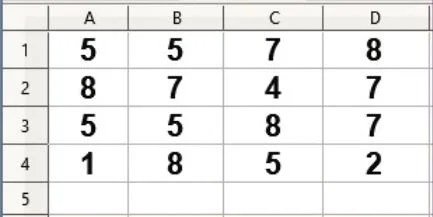Share with others

Name
Email
School Name
Consider the following sheet to find output.Q71. Write the output of =SUM (A1,B1,C1)

Q72. Write the output of =SUM(A1:C1,B2)

Q73. Write the output of =AVERAGE (A1:C1)

Q74. Write the output of =AVERAGE (A1:A3,C1:C3)

Q75. Write the output of : =MAX(A1,B1:C2)

Q76. Write the output of : =MIN(A2:C2,B3)

Q77. Write the output of : =COUNT(A1:C1,B2)

Q78. Write the output of : =COUNT(A1:A3,C1:C3)

Q79. Write the output of : =SUM(B1:C2)

Q80 Write the output of : =MIN(A1,B2,C1)

Share with others
error: Content is protected !!